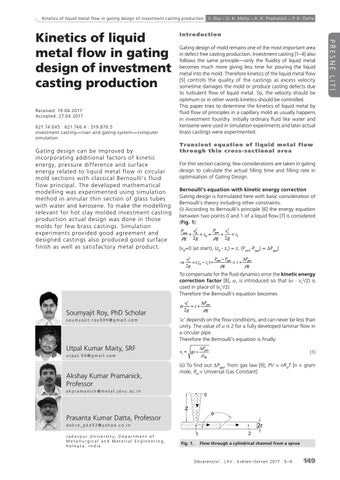Limited time offerKinetics of liquid metal flow in gating design of investment casting production

Received: 19.04.2017 Accepted: 27.04.2017 621.74.045 : 621.746.4 : 519.876.5 investment casting—riser and gating system— computer simulation

Gating design can be improved by incorporating additional fac tors of kinetic energy, pressure dif ference and sur face energy related to liquid metal flow in circular mold sec tions with classical Bernoulli’s fluid flow principal. The developed mathematical modelling was experimented using simulation method in annular thin sec tion of glass tubes with water and kerosene. To make the modelling relevant for hot clay molded investment casting produc tion ac tual design was done in those molds for few brass castings. Simulation experiment s provided good agreement and designed castings also produced good sur face finish as well as satisfac tor y metal produc t.

Introduction Gating design of mold remains one of the most important area in defect free casting production. Investment casting [1–4] also follows the same principle—only the fluidity of liquid metal becomes much more giving less time for pouring the liquid metal into the mold. Therefore kinetics of the liquid metal flow  controls the quality of the castings as excess velocity sometime damages the mold or produce casting defects due to turbulent flow of liquid metal. So, the velocity should be optimum or in other words kinetics should be controlled. This paper tries to determine the kinetics of liquid metal by fluid flow of principles in a capillary mold as usually happens in investment foundry. Initially ordinary fluid like water and kerosene were used in simulation experiments and later actual brass castings were experimented. T ra n s i e n t e q u a t i o n o f l i q u i d m e t a l f l o w through thin cross-sectional area For thin section casting, few considerations are taken in gating design to calculate the actual filling time and filling rate in optimisation of Gating Design. Bernoulli’s equation with kinetic energy correction Gating design is formulated here with basic consideration of Bernoulli’s theory including other constraints. (i) According to Bernoulli’s principle  the energy equation between P Patm v02 two points v02 and 1 of a liquid flow  is considered 1):  z0  gas  1  z1 (Fig. g 2 g g 2 g Pgas

v2

P

v2

0 z atm-Pgas) = ΔPgas] + z =(z0 - z+1) =1 z,+ (P [vatm 0=0+(at start), ρg 2 g 0 ρg 2 g 1

Patm  Pgas

v2

Pgas

1  =0 (at ( z0start),  z1 )  (z - z ) =z,z (P -P ) = ΔP ] [v 0 2g 0 g 1 atm gas g gas

∆P P −P v12 = ( z0 − z1 ) + atm gas = z + gas 2g ρg ρg

To compensate for the fluid dynamics error the kinetic energy correction factor , α, is introduced so that (α · v12 /2) is used in place of (v12 /2). Therefore the Bernoulli’s equation becomes

Soumyajit Roy, PhD Scholar soumyajit.roy009@gmail.com

Utpal Kumar Maity, SRF utpal.50@gmail.com

Akshay Kumar Pramanick, Professor

Pgas vv122   ∆Pgas = zz + α 21g  2g ρgg

‘α’ depends on the flow conditions, and can never be less than unity. The value of α is 2 for a fully developed laminar flow in a circular pipe. Therefore the Bernoulli’s equation is finally: P v1  gz  gas (1) liq

(ii) To find out ΔPgas, from gas law , PV = nRUT [n = gram mole, RU = Universal Gas Constant]

akpramanick@metal.jdvu.ac.in

Prasanta Kumar Datta, Professor dokra _ pkd52@yahoo.co.in Jadav pur U ni ver sit y, D e par tm ent of Metallurgical and Material Engineering, Kolkata, India

Fig. 1. Flow through a cylindrical channel from a sprue

S l é vá re ns t v í . L X V . k v ě te n – č e r v e n 2017 . 5 – 6

149

PŘESNÉ LITÍ

Kinetics of liquid metal flow in gating design of investment casting production

S. Roy – U. K. Maity – A. K. Pramanick – P. K. Datta